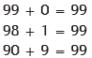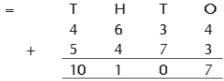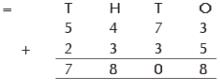Courses

Description
QUESTION: 1

Solution:
QUESTION: 2

### Find 3 different ways to make the number 99.

Solution:

3 different ways to make the number 99 areQUESTION: 3

### Tick the correct statement. Statement A : We use addition when we put two or more things together. Statement B : We use addition when we find how many things are left.

Solution:

Clearly statement is correct.

QUESTION: 4

Tick the correct statement:
Statement A : 999+0 = 999.
Statement B : 999+1 = 1+999 = 1000.

Solution:

A. 999 + 0 = 999
B. 999 + 1 = 1 + 999 = 1000
Hence both statements are correct.

QUESTION: 5

Add the following : 23512 + 28975

Solution:
QUESTION: 6

8084 + 2900

Solution:
QUESTION: 7

Tick the correct statement.

Solution:
QUESTION: 8

The annual fees of Shubhra is Rs 5372 & the annual fees of Shraddha is Rs 4352. What is their total altogether ?

Solution:
QUESTION: 9

A car travelled from town A to B and then from town B to C. The distance between towns A & B is 4364 kms. and between B & C is 5473 kms. What is the total distance travelled by car?

Solution:

Total distance travelled by car = sum of 4634 and 5473.QUESTION: 10

Swati purchased a dress for Rs 5473 & a pair of footwear of Rs 2335. What is the total amount she spent?

Solution:

Total amount = sum of 5473 and 2335